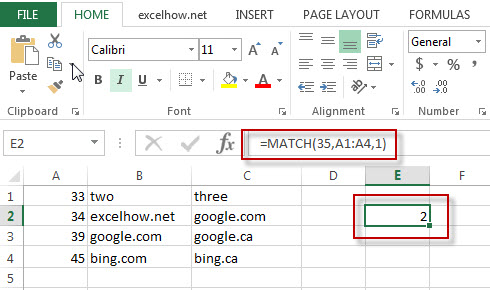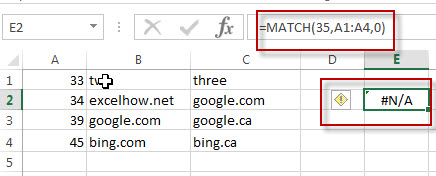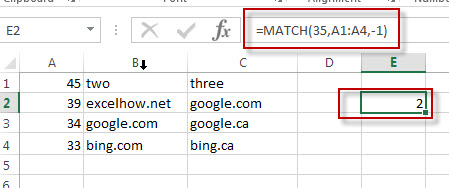Excel Match Function

This post will guide you how to use Excel MATCH function with syntax and examples in Microsoft excel.

Description

The Excel MATCH function search a value in an array and returns the position of that item.

The MATCH function is a build-in function in Microsoft Excel and it is categorized as a Lookup and Reference Function.

The MATCH function is available in Excel 2016, Excel 2013, Excel 2010, Excel 2007, Excel 2003, Excel XP, Excel 2000, Excel 2011 for Mac.

Syntax

The syntax of the MATCH function is as below:

= MATCH  (lookup_value, lookup_array, [match_type])

Where the MATCH  function arguments are:
Lookup_value -This is a required argument.  The value that you want to search.
Lookup_array – This is a required argument.  The data array that is to be searched.
Match_type – This is an optional argument.  This value can be set as: 1, 0, -1. The default value is 1.

Note:
1 – the MATCH function will search the largest value that is less than or equal to Lookup_value
0 – The MATCH function will search the first value that is exactly equal to Lookup_value
-1 – The Match function will search the smallest value that is greater than or equal to Lookup_value.

Example

The below examples will show you how to use Excel MATCH  Lookup and Reference Function .

#1 =MATCH(35,A1:A4,1)

This Excel Formula will lookup the value “35” and find the largest value that is less than or equal to “35”, it will return “2”, As the value “34” is the only  largest value that is less than to “35”. So it will return the position of value “34” in range “A1:A4”.#2 =MATCH(35,A1:A4,0)

This Formula will return the “#N/A” value, As the Match type is set to “0”, it means that the function will find the first value that is exactly equal to “35”, but it is not able to find the value “35” in “A1:A4” range.#3 =MATCH(35,A1:A4,-1)

This Formula will return “2”, if the Match type is set to “-1”, the Match function will find the smallest value that is greater than or equal to “35”in range “A1:A4”, then return its position.More Excel MATCH Formula Examples

• Finding the Max and Min value in an Alphanumeric Data
if you want to find the Maximal or minimal string value from an alphanumeric data list in the range B1:B5, you can create a formula based on the LOOKUP function and the COUNTIF function.….
• Convert Weekday Name to Number
Assuming that you have list of data B1:B7 that contain weekday names, you want to convert all weekday names to numbers.You can use a formula based on the MATCH function to achieve the result
• Find Missing Numbers in a Sequence in Excel
You can use an excel array formula based on the SMALL function, the IF function, the ISNA function, the MATCH function, and the ROW function to find missing numbers in a sequence…
• Find Closest Value or Nearest Value in a Range
You need to use an excel array formula based on the INDEX function, the MATCH function, the MIN function and the ABS function to find Closest Value or Nearest Value in a Range in Excel…
Related Posts

Extract all the matches with helper Column

With Excel's powerful functions IF, INDEX, and MATCH, we can find exactly what you're looking for with a few clicks of the mouse. This step-by-step tutorial will show how easy it is to extract data using these tools and more! ...

VLOOKUP From Another Sheet Not Working

In the previous post, you should know that how to fix or remove the #N/A error when using VLOOKUP formula to lookup value from another sheet. And this post will show you reasons why your VLOOKUP formula is not working ...

Case Sensitive Lookup with SUMPRODUCT and EXACT

Today, we will show you how to use SUMPRODUCT and EXACT to perform a case sensitive exact match. In this article, we provide a simple example to calculate bonus for employees whose names are case-sensitive. If you meet similar scenarios ...

Basic Usage of INDEX & MATCH – Case Sensitive Lookup

In Excel, INDEX function and MATCH function are often used together for retrieving data from a particular position. MATCH function is one of Excel lookup & reference functions that can perform approximate match or exact match by setting different match ...

Basic Rates Calculation by VLOOKUP Based on Weight Band

Microsoft Excel provides many functions that can execute logical test, search data, return current date and something else. They are very useful in daily work. And Excel VLOOKUP function is one of Excel most frequently used functions. It belongs to ...

Basic Grade Calculation by VLOOKUP Function – Approximate Match

In Excel, except combination INDEX+MATCH, we can also apply other functions to search data, for example VLOOKUP function. Like MATCH function, VLOOKUP function is one of Excel lookup & reference functions that can perform approximate match or exact match by ...

Basic Discount Calculation with VLOOKUP Function

In Excel, except combination INDEX+MATCH, we can also apply other functions to search data, for example VLOOKUP function. Like MATCH function, VLOOKUP function is one of Excel lookup & reference functions that can perform approximate match or exact match by ...

Basic Usage of INDEX & MATCH – Exact Match

In Excel, INDEX function and MATCH function are often used together for returning value or cell reference or range reference from specified position. And MATCH function is one of Excel lookup & reference functions that can perform approximate match or ...

Basic Usage of INDEX & MATCH – Approximate Match

In Excel, INDEX function and MATCH function are often used together for returning value or cell reference or range reference from specified position. And MATCH function is one of Excel lookup & reference functions that can perform approximate match by ...

Approximate Match with Multiple Criteria by INDEX & MATCH

In Excel, INDEX function and MATCH function are often used together for returning data from specific position. And MATCH function is one of Excel lookup & reference functions that can return approximate value by setting match type. Above all, through ...

Sidebar